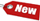Browse Accounting Lessons HereAccounting Terms & DefinitionsAccounting for Merchandising ActivitiesDebits and Credits (Double Entry Accounting)Business Valuation FormulasTime Value of Money & Present/Future ValuesComplex Debt & Equity InstrumentsCommon Stock & Shareholder's EquityAccounting & Finance RatiosValuing Common StockCorporate Income TaxesLower of Cost or Market (LCM) & Inventory ValuationChart of Accounts & BookkeepingBonds Payable & Long Term LiabilitiesCapital AssetsGAAP, Accrual & Cash Accounting, Information Commodity, Internal Controls & MaterialityWhat category of browser are you on this website? Accounting student (homework help) Finance professor (university research) Accounting manager (at work) Other Explore Careers in Accounting and FinanceVisit our section on Careers in Accounting & Finance to explore vast opportunities in this industry.

Dividend Growth Model - How to Value Common Stock with a Constant Dividend and Steady Growth

If the dividend grows at a steady rate, we do not need to forecast an infinite number of future dividends; however we just need to come up with a single growth rate which is a lot simpler. Taking D0 to be the dividend just paid and g to be the constant growth rate, the value of one share of stock can be simply written as:

 P0 = [(D1 / (1 + r)1)] + [(D2 / (1 + r)2)] + [(D3 / (1 + r)3)]

This can be cut down to the following:

 P0 = [D0 x (1 + g)] / (r – g) = (D1) / (r – g)

We have therefore just derived the dividend growth model which is a model that determines the current price or value of a share of stock as its dividend next period divided by the discount rate minus the dividend growth rate.

Example

Consider a case where the current dividend payout is \$1.80 and the rate of return required is 15% while the constant growth rate is 5%. What will the current price of the stock be?

 P0 = [D0 x (1 + g)] / (r – g) P0 = [\$1.80 x (1 + 0.05) / (0.15 – 0.05) P0 = [\$1.80 x (1.05) / (0.1) P0 = \$1.89 / 0.1 P0 = \$18.90

Bank of America Dividend Growth Model Application - ExampleBank of America announces its next dividend will be \$2 a share and from research we know that investors typically require a 14% annual rate of return from American banks, thus this will be the required rate of return. The bank increases its dividend at a steady rate of 8% a year. Based on the dividend growth model, what is the value of the Bank of America stock today? What is the value in 4 years?

a) Since we are already given the next dividend as \$2 per share, we will not multiply D1 with (1 + g) as it is given as \$2. Having said this, the dividend growth formula we will use is:

 P0 = D1 / (r – g) P0 = 2 / (0.14 – 0.08) P0 = 2 / 0.06 P0 = \$33.33

b) Since we already know the dividend in one year, the dividend in four years is equal to:

 P0 = D1 / (r – g) P0 = 2 / (0.14 – 0.08) P0 = 2 / 0.06 P0 = \$33.33

Therefore, the price of the stock in four years will be:

 P4 = [(D4 x (1 + g)] / (r – g) P4 = (\$2.52 x (1 + 0.08) / (0.14 – 0.08) P4 = (\$2.52 x (1.08) / 0.06 P4 = 2.7216 / 0.06 P4 = \$45.36

Dividend Growth Sample Problems – Example 1

ABC Company has just paid a \$1.5 dividend and dividends are expected to grow at 5% per year. Return on shareholder’s equity is 12%. What is the stock price?

 P0 = [(D0 (1 + g) / (re – g)] P0 = 1.5 (1.05) / (0.12 – 0.05) P0 = 1.575 / 0.07 P0 = \$22.50 (Current stock price)

Dividend Growth Sample Problems – Example 2

ABC Company is a declining company and dividends are expected to decrease by 4% a year. Next year’s dividends are expected to come in at \$2 per share and the required shareholder return is 8%.

a) What is the current price of the stock?

 P0 = D1 / (Re – g) P0 = \$2 / (0.08 - - (0.04)) P0 = \$2 / 0.12 P0 = \$16.67